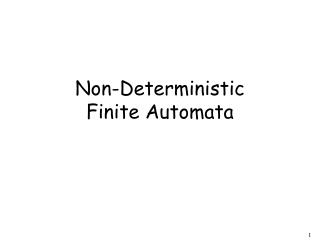DownloadDownload PresentationNon-Deterministic Finite Automata

# Non-Deterministic Finite Automata

Télécharger la présentation## Non-Deterministic Finite Automata

- - - - - - - - - - - - - - - - - - - - - - - - - - - E N D - - - - - - - - - - - - - - - - - - - - - - - - - - -
##### Presentation Transcript

1. Non-Deterministic Finite Automata

2. Nondeterministic Finite Automaton (NFA) Alphabet =

3. Alphabet = Two choices

4. Alphabet = Two choices No transition No transition

5. First Choice

6. First Choice

7. First Choice

8. First Choice All input is consumed “accept”

9. Second Choice

10. Second Choice

11. Second Choice No transition: the automaton hangs

12. Second Choice Input cannot be consumed “reject”

13. An NFA accepts a string: when there is a computation of the NFA that accepts the string There is a computation: all the input is consumed and the automaton is in an accepting state

14. Example is accepted by the NFA: “accept” “reject” because this computation accepts

15. Rejection example

16. First Choice

17. First Choice “reject”

18. Second Choice

19. Second Choice

20. Second Choice “reject”

21. An NFA rejects a string: when there is no computation of the NFA that accepts the string. For each computation: • All the input is consumed and the • automaton is in a non final state OR • The input cannot be consumed

22. Example is rejected by the NFA: “reject” “reject” All possible computations lead to rejection

23. Rejection example

24. First Choice

25. First Choice No transition: the automaton hangs

26. First Choice Input cannot be consumed “reject”

27. Second Choice

28. Second Choice

29. Second Choice No transition: the automaton hangs

30. Second Choice Input cannot be consumed “reject”

31. is rejected by the NFA: “reject” “reject” All possible computations lead to rejection

32. Language accepted:

33. Lambda Transitions

35. all input is consumed “accept” String is accepted

36. Rejection Example